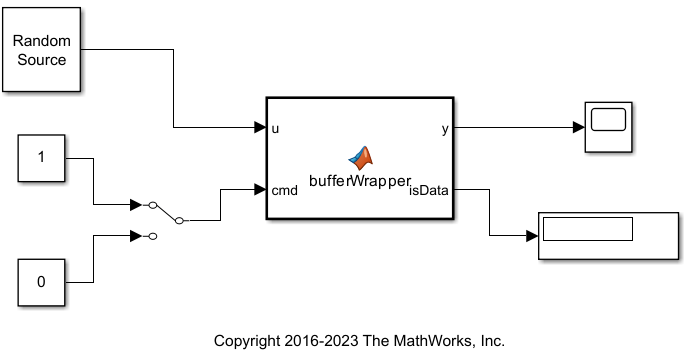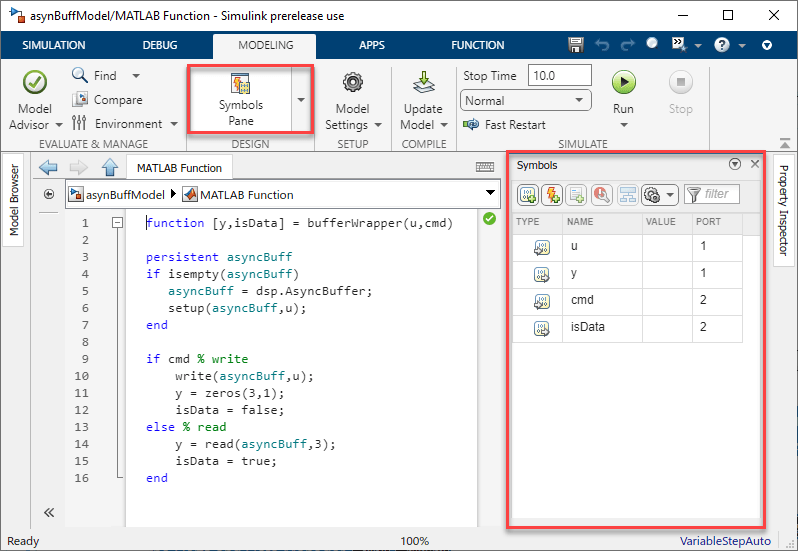# Why Does Reading Data from the dsp.AsyncBuffer Object Give a Dimension Mismatch Error in MATLAB Function Block?

If you are reading data from an asynchronous buffer inside a MATLAB Function (Simulink) block, the block throws a dimension mismatch error if the output of the `read` function is not specified to be a variable-size signal.Here is the `bufferWrapper` function that contains the algorithm inside the MATLAB Function block. When input on the `cmd` port is 1, the `dsp.AsyncBuffer` object writes the data input `u` to the buffer. When input on the `cmd` port is 0, the object reads data from the buffer.

`type bufferWrapper.m`
```function [y,isData] = bufferWrapper(u,cmd) persistent asyncBuff if isempty(asyncBuff) asyncBuff = dsp.AsyncBuffer; setup(asyncBuff,u); end if cmd % write write(asyncBuff,u); y = zeros(3,1); isData = false; else % read y = read(asyncBuff,3); isData = true; end ```

You must initialize the buffer by calling either `write` or `setup` before the first call to `read`.

During the write operation, the first output `y` is `zeros(3,1)` and the second output `isData` is 0. During the read operation, `y` is the data in the buffer and `isData` is 1.

Run the model and the following error occurs.The output of `read(asyncBuff,3)` is variable sized. The output is variable sized because the size of the signal output by the `read` function depends on the input arguments to `read`. To resolve this error, set `y` as a variable-size signal and specify the upper bound.

In the MATLAB Function block Editor, click Modeling tab. In the Design section, click Symbols Pane. The Symbols pane opens on the right.Right-click `y` and select `Inspect`.Property Inspector window opens. In the Advanced section, select the Variable size check box. Specify the Size to 3 since that is the size of the data the MATLAB function writes to the variable `y`.Save and run the model. The error disappears. View the output `y` in the Time Scope.With `cmd` = 0, no data is written into the buffer. Therefore, the output is 0. To write the input data u to the buffer, set `cmd` = 1. After you write some data, if you change `cmd` back to 0, the Time Scope output changes to the following.